Computer Science

# Introduction to images## Understanding images

### Pixels

• Digital images consist of pixels
• A pixel is a small square, showing a single color

Download and view pebbles.jpeg with an image viewer, zoom in, and see the pixels it uses.

### RGB

• each pixel can be controlled using a combination of red, green and blue
• each color ranges from a value of 0 (minimum) to 255 (maximum)
• these colors mix to form the color of the pixel
• visit rgb-explorer to see color mixing in action

## The byuimage library

• code we have written to make it easier to work with images
• similar to byubit, but in for images
• visit your CS110 conda environment, and in a PyCharm terminal install this:
``pip install byuimage``

``````from byuimage import Image

image = Image('flower.jpeg')
image.show()
``````

### Looping over the pixels

• the pixels in the image are a collection
• this collection is ordered -> they go from left to right and top to bottom
• (0,0) -> (1,0) -> (2,0) …
• (0,1) -> (1,1) -> (2,1) …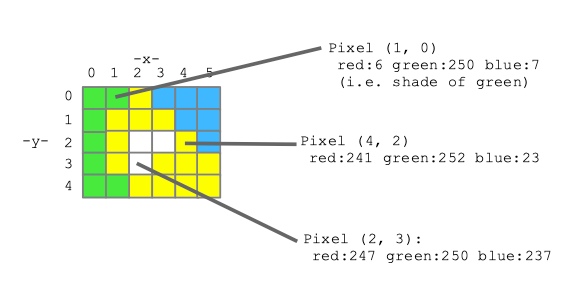### Looping over the pixels and changing them

``````for pixel in image:
...``````
• In this loop, we now have a `pixel` variable that is set to the current pixel (fro top to bottom, left to right)
• pixel.green allows us to change the green value of the variable
• the same for pixel.green and pixel.blue
``````for pixel in image
pixel.green = 50``````

## Modifying an image

• Let’s set the blue and green values in each pixel of the image to 0
• this will leave just the red values — the “red channel” of the image
``````image = Image("flower.jpeg")
for pixel in image:
pixel.green = 0
pixel.blue = 0
image.show()``````

### Looping over the pixels

• each time through the for loop, the `pixel` variable is set to the current pixel
• the variable could be called `truck`, but we like our variables to make sense
``````image = Image("flower.jpeg")
for truck in image:
truck.green = 0
truck.blue = 0
image.show()``````
``````image = Image("flower.jpeg")
for pixel in image:
pixel.green = 0
pixel.blue = 0
image.show()``````

### Play around with the code

• what about a green channel?
• making all pixels black?
• inverting the pixels?
• swapping red, green, and blue?
``````image = Image("flower.jpeg")
for pixel in image:
pixel.green = 0
pixel.blue = 0
image.show()``````

### Invert the pixels

``````image = Image("flower.jpeg")
for pixel in image:
pixel.red = 255 - pixel.red
pixel.green = 255 - pixel.green
pixel.blue = 255 - pixel.blue
image.show()``````

### Swap red, green, blue colors

``````image = Image("flower.jpeg")
for pixel in image:
red = pixel.red
pixel.red = pixel.green
pixel.green = pixel.blue
pixel.blue = red
image.show()``````

## Image functions

### Write a function for red channel

``````def red_channel(filename):
image = Image(filename)
for pixel in image:
pixel.green = 0
pixel.blue = 0
return image

red_flower = red_channel("flower.jpeg")
red_flower.show()``````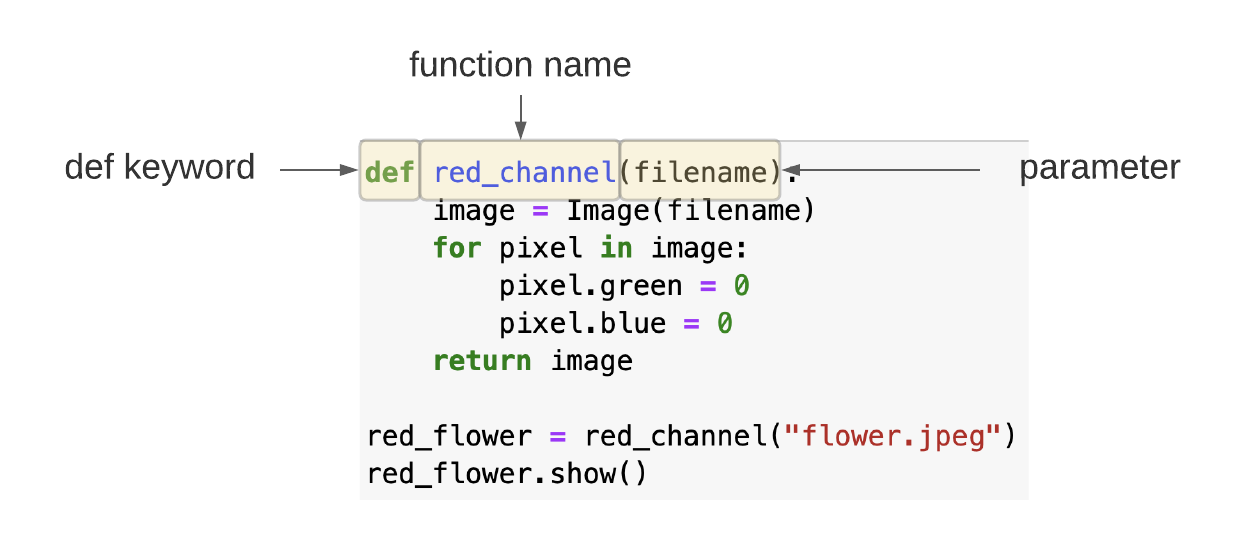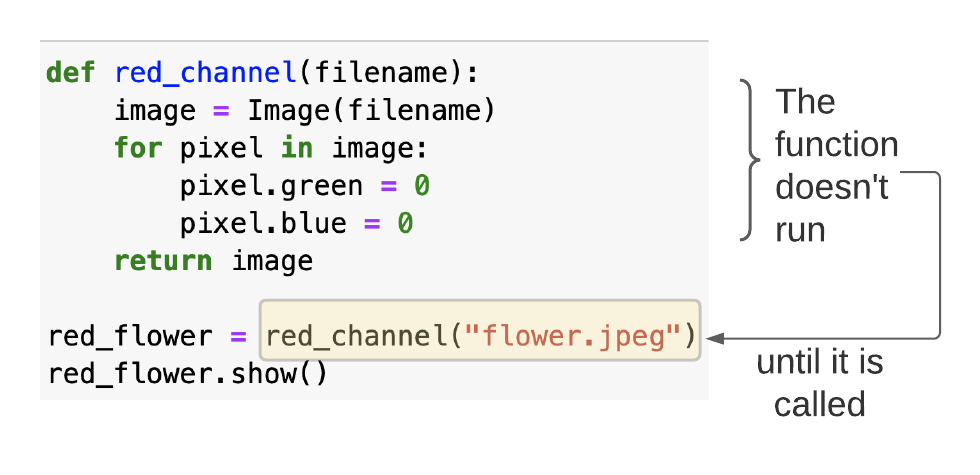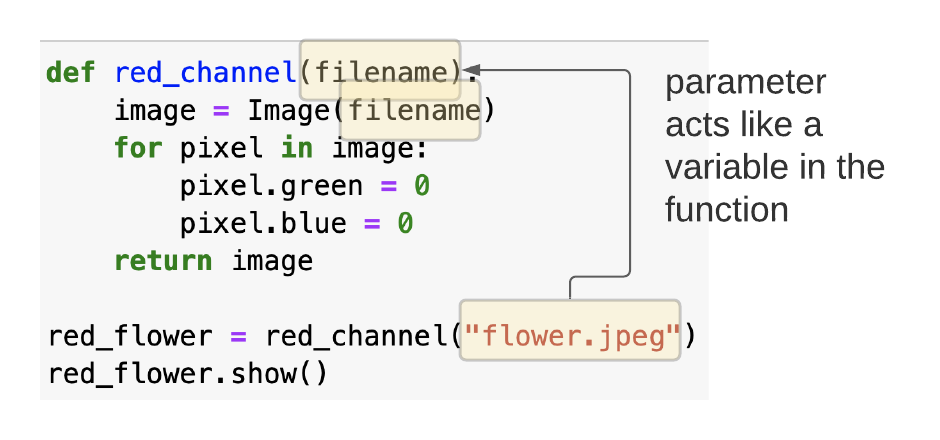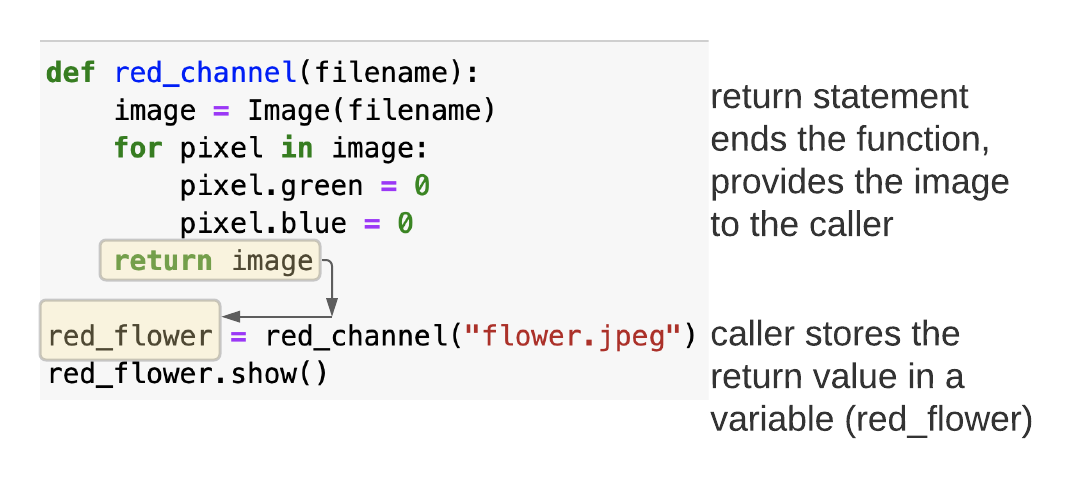### How many variables are used in this code?

``````def red_channel(filename):
image = Image(filename)
for pixel in image:
pixel.green = 0
pixel.blue = 0
return image

red_flower = red_channel("flower.jpeg")
red_flower.show()``````
• filename — function parameter becomes a variable inside the function
• image — variable inside the function
• red_flower — variable outside the function

### Darkening an image

``````def darken(filename):
image = Image(filename)
for pixel in image:
pixel.red = pixel.red * 0.5
pixel.green = pixel.green * 0.5
pixel.blue = pixel.blue * 0.5
return image

darkened = darken("flower.jpeg")
darkened.show()
``````

## if statements inside a while loop

### Remove the green

Change pixels that are green to black

``````from byuimage import Image

def remove_green(filename):
image = Image(filename)
image.show()
for pixel in image:
if pixel.green > 100:
pixel.red = 0
pixel.blue = 0
pixel.green = 0
return image

img = remove_green("flower.jpeg")
img.show()``````

### Remove the green

Use a better method to identify green pixels: take the average of red, blue, and green and a green pixel has its green value bigger than the average

``````def remove_green(filename):
image = Image(filename)
image.show()
for pixel in image:
average = (pixel.red + pixel.blue + pixel.green) / 3
if pixel.green > average:
pixel.red = 0
pixel.blue = 0
pixel.green = 0
return image

img = remove_green("flower.jpeg")
img.show()``````